# Prediction, Bootstrap and Simulation for Nonlinear Models

#### 2021-04-21

Preliminaries: first, we need to load required libraries.

library(ggplot2)
library(nlraa)
library(car)
## Loading required package: carData
library(nlme)

# Bootstrapping

The bootstrap is a technique in statistics which consists of resampling the observed data in order to create an empirical distribution of some statistic (Fox and Weisberg, 2012, 2017). This is often done to evaluate the assumptions of the model or to derive empirical distributions which are difficult to approximate. For nonlinear models, bootstrapping can be useful because often questions arise that a typical analysis does not answer. In many instances the distributions of parameters from a nonlinear model are in fact normal and standard approximations work well, in other instances, however, this is not the case.

In the following sections I will illustrate the use of the bootstrap for nonlinear model (nls), generalized nonlinear models (gnls) and nonlinear mixed models (nlme). In another section I will illustrate the ability to simulate from nonlinear (mixed) models

## Nonlinear models

The first type of models illustrated here are nonlinear models for which we make standard assumptions for error distributions and there are no random effects.

### Simple example

As a simple example, we can use the dataset ‘barley’ in the ‘nlraa’ package. This represents the response of barley yield to different doses of fertilizer over several seasons. We will ignore the effect of ‘year’ in this section.

data(barley, package = "nlraa")

## Quick visualization
ggplot(data = barley, aes(x = NF, y = yield)) + geom_point()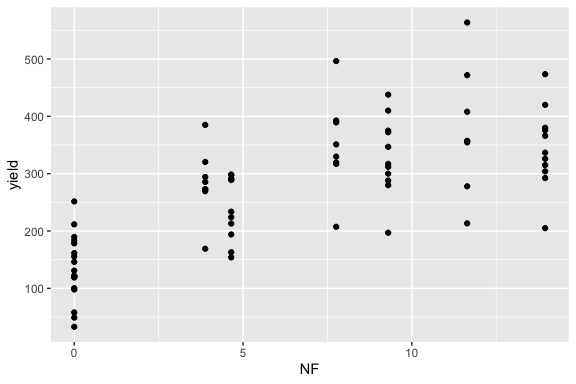We can fit a very simple model known as the linear-plateau.

## Linear-plateau model
## The function SSlinp is in the 'nlraa' package
fit.lp <- nls(yield ~ SSlinp(NF, a, b, xs), data = barley)

## Visualize data and fit
ggplot(data = barley, aes(x = NF, y = yield)) +
geom_point() +
geom_line(aes(y = fitted(fit.lp)))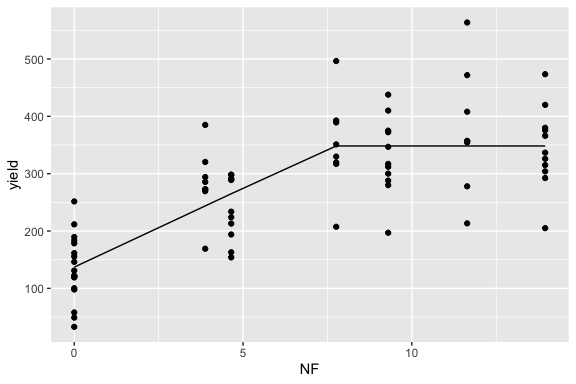At this point several questions might arise:

1. What are the confidence intervals for the model parameters?
2. How do we describe the uncertainty around the fitted values of the model?
3. What is the estimate and confidence interval for the asymptote?

### Confidence interval for model parameters

The confidence intervals for the model parameters can be derived using a method called profiling. The generic function ‘confint’ can be used which invokes ‘confint.nls’ from the ‘MASS’ package.

confint(fit.lp)
## Waiting for profiling to be done...
##          2.5%     97.5%
## a  104.821725 167.73660
## b   19.937411  38.00267
## xs   5.849474  12.23099

For nonlinear models this method is preferred to simple confidence intervals that would directly use the standard error of the model parameters. In many cases, there is good agreement between the two, but this is not always the case and it can be informative to compare both methods. Those standard errors can be obtained using the ‘summary’ function, along with hypothesis testing.

summary(fit.lp)
##
## Formula: yield ~ SSlinp(NF, a, b, xs)
##
## Parameters:
##    Estimate Std. Error t value Pr(>|t|)
## a   137.067     16.179   8.472 1.83e-12 ***
## b    27.501      5.269   5.219 1.62e-06 ***
## xs    7.682      1.211   6.342 1.68e-08 ***
## ---
## Signif. codes:  0 '***' 0.001 '**' 0.01 '*' 0.05 '.' 0.1 ' ' 1
##
## Residual standard error: 70.79 on 73 degrees of freedom
##
## Number of iterations to convergence: 2
## Achieved convergence tolerance: 6.548e-16

Some interesting plots can be produced which illustrate the symmetry (or lack thereof) of the distribution for the model parameters. Profile plots which show large departures from normality would indicate that the normal approximation for the confidence intervals might not be the best choice.

## For the intercept
plot(profile(fit.lp, "a"))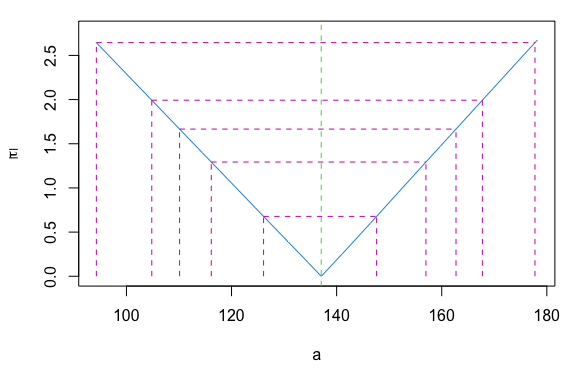## This one is fairly symetric and the normal approximation is reasonable
plot(profile(fit.lp, "b"))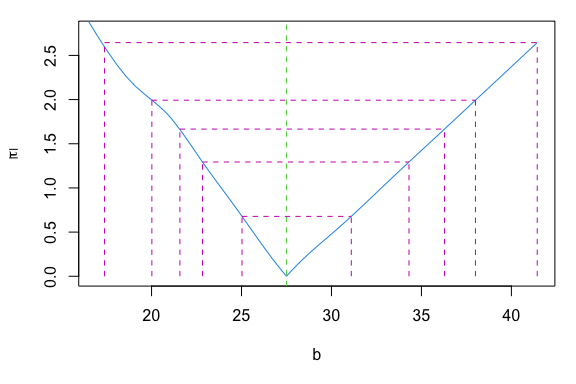plot(profile(fit.lp, "xs"))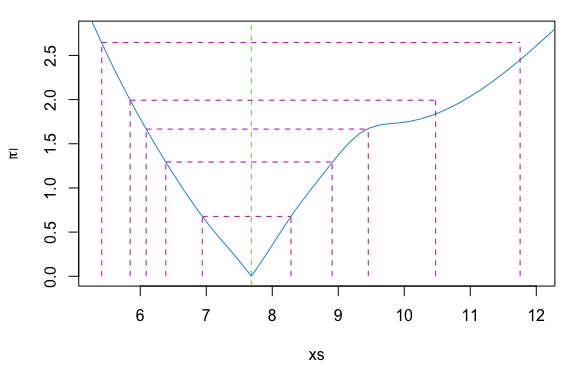## These last parameters are less symetrical

Being able to see the whole profile for a parameter can be interesting in terms of understanding its behavior. For example, in this model, which has a ‘break-point’, we would not expect all the parameters to have identical shaped profiles and that is illustrated above for parameter ‘xs’.

In this case, bootstraping is an alternative to computing the confidence intervals and it can be used as a way to double-check the previous results, but it can also be used when profiling fails. A couple of functions which can perform bootstraping for nonlinear models are ‘boot_nls’ in the ‘nlraa’ pacakge or the ‘Boot’ in the ‘car’ pacakge.

## psim = 3 just adds residuals and does not resample parameters
fit.lp.Bt <- boot_nls(fit.lp, psim = 3)
## Number of times model fit did not converge 174 out of 999
## Or you can use the Boot function in the car package

In this case, this function uses the base ‘boot’ package under the hood and it returns an object of that class. This also allows for other options such as defining a function as a combination of model parameters, and the use of more than one core to speed up computation. In this case, since we are also interested in the asymptote, which is ‘a + b * xs’, we can obtain confidence intervals for this parameter by doing the following.

## I'm using Boot in the 'car' package here, but it is similar to 'boot_nls'
fn <- function(x) coef(x) + coef(x) * coef(x)
fit.lp.Bt.asymp <- Boot(fit.lp, f = fn, labels = "asymptote")
##
##  Number of bootstraps was 792 out of 999 attempted
confint(fit.lp.Bt.asymp)
## Bootstrap bca confidence intervals
##
##              2.5 %   97.5 %
## asymptote 323.1819 379.0912
hist(fit.lp.Bt.asymp)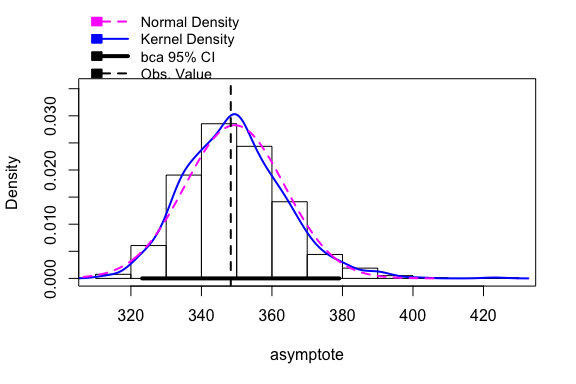The bootstrap method takes a few seconds here, but it can be computationally much more demanding in larger problems since it re-fits the model many, many times. An alternative is to use the delta method, for which there is a function in the ‘car’ pacakge. The delta method has the advantage of being fast and the disadvantage that it makes the assumption of a normal distribution. In this case the lower bound for the confidence interval is similar to the one obtained with the bootstrap, but the upper bound for the confidence interval is somewhat higher using the bootstrap (381 vs. 370). Since the bootstrap method makes fewer assumptions it is probably the better one to report.

fit.lp.Dlt.asymp <- deltaMethod(fit.lp, "a + b * xs")
fit.lp.Dlt.asymp
##            Estimate      SE   2.5 % 97.5 %
## a + b * xs  348.326  11.484 325.817 370.84

In order to answer our second question above related to the uncertainty around the fitted values we could plug-in the values from the bootstrap sampling and obtain different regression lines.

## The object 't' in the bootstrap run has
## the parameter estimate values
## First remove missing values
fit.lp.Bt.prms <- na.omit(fit.lp.Bt$t) nrb <- length(unique(barley$NF))
nrp <- nrow(fit.lp.Bt.prms)

## Set up an empty data.frame
prd.dat <- data.frame(i = as.factor(rep(1:nrp, each = nrb)), NF = rep(unique(barley$NF), nrp), prd = NA) ## A simple loop can be used to run the model multiple times for(i in 1:nrp){ a.i <- fit.lp.Bt.prms[i,1] b.i <- fit.lp.Bt.prms[i,2] xs.i <- fit.lp.Bt.prms[i,3] prd.dat[c(1 + (nrb*(i - 1))):c(i * nrb),3] <- linp(unique(barley$NF), a.i, b.i, xs.i)
}

## Plot the data with the original fit and the uncertainty
ggplot() +
geom_line(data = prd.dat, aes(x = NF, y = prd, group = i),
color = "gray", alpha = 0.2) +
geom_line(data = barley, aes(x = NF, y = fitted(fit.lp))) +
geom_point(data = barley, aes(x = NF, y = yield)) +
ylab("Yield") + xlab("Nitrogen rate") +
ggtitle("Using results from Boot \n and plug-in into linp")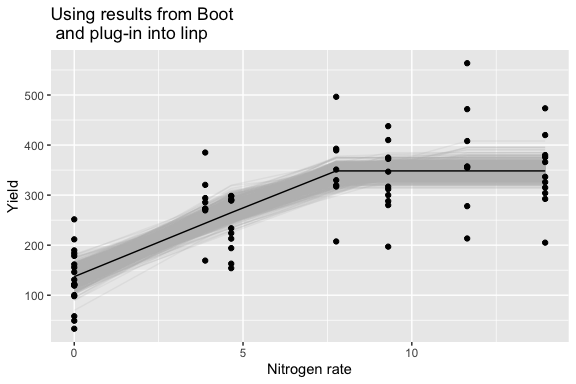The previous graph shows the black line with the original fit and the variability in the fitted values due to the resampling generated during the bootstrap process. The function ‘predict.nls’ at the moment ignores the arguments ‘se.fit’ and ‘interval’ which means that this functionality is not available in the base ‘stats’ package. (There is an alternative approach in the ‘propagate’ package - see references.)

So we could use the quantiles of ‘prd.dat’ object above to derive confidence intervals for the regression line. The previous example is an attempt to make it clear how the uncertainty could be displayed. Equivalently, we can use ‘Boot’ for this purpose, but with some effort manipulating the data.

fn2 <- function(x) predict(x, newdata = data.frame(NF = 0:14))
fit.lp.Bt2 <- Boot(fit.lp, fn2)
##
##  Number of bootstraps was 819 out of 999 attempted
fttd <- na.omit(fit.lp.Bt2$t) prds <- c(t(fttd)) ndat <- data.frame(i = as.factor(rep(1:nrow(fttd), each = ncol(fttd))), NF = rep(0:14, nrow(fttd))) ndat$prd <- prds

## Essentially the same graph as the one above
ggplot() +
geom_line(data = ndat, aes(x = NF, y = prd, group = i),
color = "gray", alpha = 0.2) +
geom_line(data = barley, aes(x = NF, y = fitted(fit.lp))) +
geom_point(data = barley, aes(x = NF, y = yield)) +
ylab("Yield") + xlab("Nitrogen rate")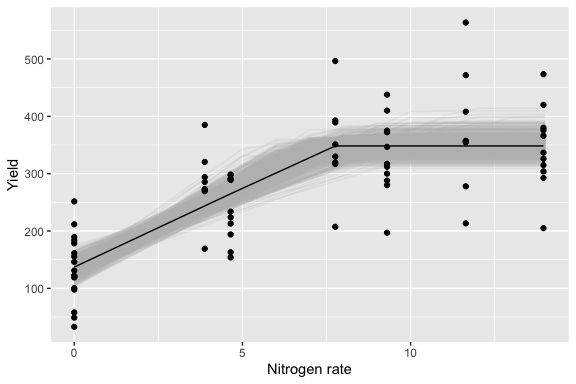## Bootstrapping generalized nonlinear models

Implementing bootstrap for more complex models takes extra work. For this, I’m taking the approach of sampling from the vector of fixed parameters and also bootstrapping the standardized residuals for ‘gnls’ and ‘nlme’ objects. (This methodology can be improved in the future.) This takes advantage that we assume that the residuals are normally distributed.

As a first example, we can compare the bootstrapped confidence intervals with the ones obtained by ‘intervals’. Note: I’m running this only a few hundred times in the examples below for efficiency, but it is a good practice to run these a few thousand times.

set.seed(101)
## Simplify the dataset to make the set up simpler
barley2 <- subset(barley, year < 1974)

fit.lp.gnls2 <- gnls(yield ~ SSlinp(NF, a, b, xs), data = barley2)

intervals(fit.lp.gnls2)
## Approximate 95% confidence intervals
##
##  Coefficients:
##        lower       est.      upper
## a  138.79981 176.800000 214.800186
## b   13.75248  27.603093  41.453706
## xs   3.64251   6.174127   8.705744
## attr(,"label")
##  "Coefficients:"
##
##  Residual standard error:
##    lower     est.    upper
## 25.50341 35.17935 56.67543
## Compare this to the bootstrapping approach
## R = 200 is too low for bootstrap, this is for illustration only
fit.lp.gnls2.bt <- boot_nlme(fit.lp.gnls2, R = 200)
## Number of times model fit did not converge 22 out of 200
summary(fit.lp.gnls2.bt)
##
## Number of bootstrap replications R = 178
##   original  bootBias  bootSE  bootMed
## 1 176.8000 -1.881489 23.1895 174.7183
## 2  27.6031  1.095069  7.4412  28.4382
## 3   6.1741 -0.072778  1.3834   5.9537
confint(fit.lp.gnls2.bt, type = "perc")
## Bootstrap percent confidence intervals
##
##        2.5 %     97.5 %
## 1 125.320034 224.479385
## 2  14.440280  42.522212
## 3   4.040724   9.591933

The confidence intervals obtained by bootstrap are wider (as expected) than the ones obtained using intervals because they consider the uncertainty in the parameters of the nonlinear model. The next example shows a slightly more complex model in which we introduce the (fixed) effect of year for a subset of the data.

set.seed(101)
barley2$year.f <- as.factor(barley2$year)

cfs <- coef(fit.lp.gnls2)

fit.lp.gnls3 <- update(fit.lp.gnls2,
params = list(a + b + xs ~ year.f),
start = c(cfs, 0, 0, 0,
cfs, 0, 0, 0,
cfs, 0, 0, 0))

## This bootstraps the vector of parameters
fit.lp.gnls3.bt <- boot_nlme(fit.lp.gnls3, R = 300)
## Number of times model fit did not converge 152 out of 300
confint(fit.lp.gnls3.bt, type = "perc")
## Bootstrap percent confidence intervals
##
##          2.5 %     97.5 %
## 1  111.1375192 196.119143
## 2  -45.3383112  86.644389
## 3  -52.9212287  65.101962
## 4   -0.5059473 114.993794
## 5   14.3750448  41.574802
## 6  -20.9463834  15.697041
## 7  -17.7796470  16.955886
## 8  -18.1083851  16.965825
## 9    4.4735481   9.074686
## 10  -2.6628309   4.960814
## 11  -4.1799869   1.682101
## 12  -2.8113050   3.862596
hist(fit.lp.gnls3.bt, 1, ci = "perc")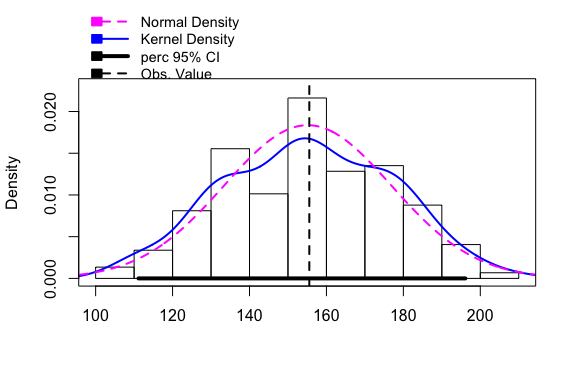This is simply to illustrate the use of bootstrapping for a ‘gnls’ object, which is something that function ‘car::Boot’ does not seem to be able to handle (the deltaMethod function also fails for this type of model, because it cannot handle the names in the vector of coefficients which uses periods).

## Bootstrapping nonlinear mixed models

For illustration, I will continue to use the barley example, but this time the model is fitted to each year individually and then a nonlinear mixed model which assumes a diagonal matrix for the random effects (for simplicity). In this case we want an esimtate of the confidence interval for the asymptote which is not an explicit parameter but rather a combination ‘a + b * xs’ of the three parameters.

set.seed(101)
barley$year.f <- as.factor(barley$year)

barleyG <- groupedData(yield ~ NF | year.f, data = barley)

fitL.bar <- nlsList(yield ~ SSlinp(NF, a, b, xs), data = barleyG)

fit.bar.nlme <- nlme(fitL.bar, random = pdDiag(a + b + xs ~ 1))

## Confidence intervals of the model fixed parameters
intervals(fit.bar.nlme, which = "fixed")
## Approximate 95% confidence intervals
##
##  Fixed effects:
##         lower       est.     upper
## a  109.212647 134.663569 160.11449
## b   23.970656  27.939486  31.90832
## xs   6.823868   7.671139   8.51841
## attr(,"label")
##  "Fixed effects:"
## Function which computes the asymptote
fna <- function(x) fixef(x) + fixef(x) * fixef(x)

## Bootstrap the model for the asymptote
fit.bar.nlme.bt <- boot_nlme(fit.bar.nlme, f = fna, R = 200)
## Number of times model fit did not converge 90 out of 200
confint(fit.bar.nlme.bt, type = "perc")
## Bootstrap percent confidence intervals
##
##     2.5 %   97.5 %
## 1 306.707 382.6985
hist(fit.bar.nlme.bt, ci = "perc")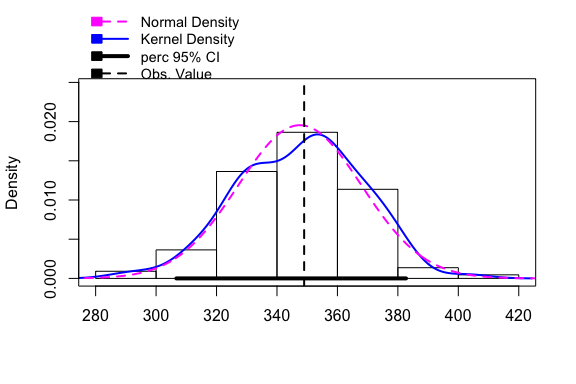For this model the estimate for the asymptote is 349, but from the model fit we do not get a direct confidence interval. Bootstrapping makes this easy (but it takes a bit of time) and it results in 307, 383.

## Confidence bands for generalized nonlinear models

For this example I will use the Orange dataset. The goal here is to place confidence bands around the mean response function.

data(Orange)

## This fits a model which considers the fact that
## the variance typically increases as the fitted
## values increase
fitg <- gnls(circumference ~ SSlogis(age, Asym, xmid, scal),
data = Orange, weights = varPower())

## Here we use bootstrapping to investigate
## the uncertainty around the fitted values
fitg.bt1 <- boot_nlme(fitg, fitted, psim = 1, R = 300)
## Number of times model fit did not converge 3 out of 300
## Compute 90% quantile confidence bands
lwr1.q <- apply(t(fitg.bt1$t), 1, quantile, probs = 0.05, na.rm = TRUE) upr1.q <- apply(t(fitg.bt1$t), 1, quantile, probs = 0.95, na.rm = TRUE)

ggplot() +
geom_point(data = Orange, aes(x = age, y = circumference)) +
geom_line(data = Orange, aes(x = age, y = fitted(fitg))) +
geom_ribbon(aes(x = Orange$age, ymin = lwr1.q, ymax = upr1.q), fill = "purple", alpha = 0.2) + ggtitle("Orange fit using the logistic: \n 90% confidence band for the mean function")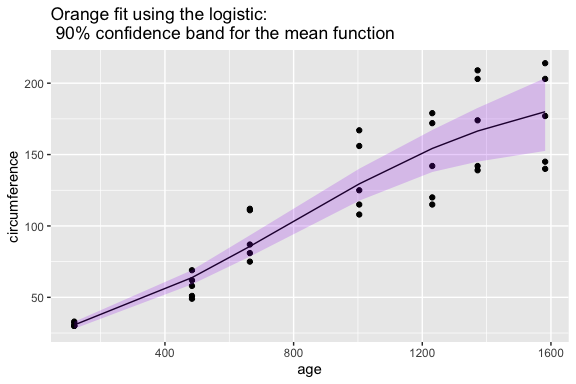# Simulation Here I will continue with the Orange dataset and illustrate different types of simulation which are relevant depending on what the inference scope is. This simulation is, in fact, used inside of the bootstrap function above; it is a necessary step for bootstrap for these type of models. ## Fitted values The first level of predictions we can generate for the Orange dataset are at the ‘population’ level. These are similar to the fitted values for the ‘gnls’ case above. Notice that we do not have the option of requesting standard errors for the predicitons for this model either. fmoL <- nlsList(circumference ~ SSlogis(age, Asym, xmid, scal), data = Orange) fmo <- nlme(fmoL, random = pdDiag(Asym + xmid + scal ~ 1)) ## Just one simulation, because with psim = 0 and level = 0, we are ## computing the predicted values at level = 0 (?predict.nlme) sim00 <- simulate_nlme(fmo, nsim = 1, psim = 0, level = 0) dat00 <- cbind(Orange, prd = as.vector(sim00)) ggplot(data = dat00) + geom_point(aes(x = age, y = circumference)) + geom_line(aes(x = age, y = prd)) + ggtitle("psim = 0, level = 0")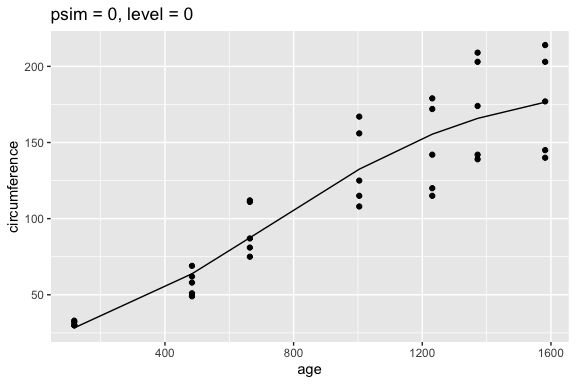One approach we can use to assess the uncertainty in the response would be to sample from the vector of parameters and the associated variance-covariance matrix. In this case, we could do many realizations, but I’ll keep it to just 100. A frequentist interpretation of the bands below would be that in repeated sampling from this same population we would expect that the true relationship will be within this band with a specified probability. One way to compute these bands (which I’m not doing below), would be to calculate the quantiles of the empirical distribution. These could be called confidence bands, which would be equivalent to the ‘interval = confidence’ in a linear model (?predict.lm). sdat10 <- simulate_nlme(fmo, nsim = 100, psim = 1, level = 0, value = "data.frame") ggplot(data = sdat10) + geom_line(aes(x = age, y = sim.y, group = ii), color = "gray", alpha = 0.5) + geom_point(aes(x = age, y = circumference)) + ggtitle("psim = 1, level = 0") + ylab("circumference")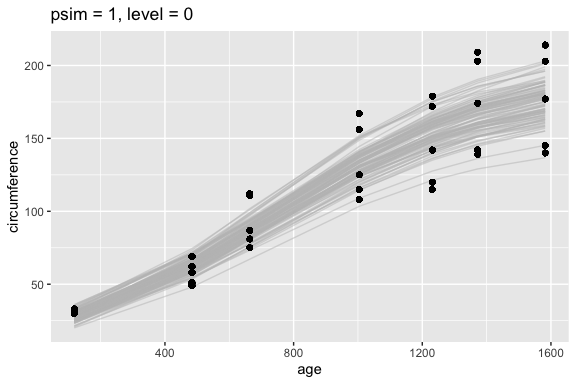In this case, each tree is an ‘individual’ and the previous one is a population level confidence. Next, we would like to produce individual level ‘prediction’ bands. This is, if we were able to resample from a population with a similar structure and if we wanted to have bands that include the true relationship between circumference and age for these trees, we could produce the confidence bands from the quantiles of the empirical distribution of the lines in the figure below (we would need a few thousand samples in order to do this). sdat11 <- simulate_nlme(fmo, nsim = 100, psim = 1, level = 1, value = "data.frame") ## We need a tree simulation ID ## for plotting sdat11$Tree_ID <- with(sdat11, paste0(Tree,"_",ii))

ggplot(data = sdat11) +
facet_wrap(~ Tree) +
geom_line(aes(x = age, y = sim.y, color = Tree, group = Tree_ID),
alpha = 0.5) +
geom_point(aes(x = age, y = circumference)) +
ggtitle("psim = 1, level = 1") +
ylab("circumference") +
theme(legend.position = "none")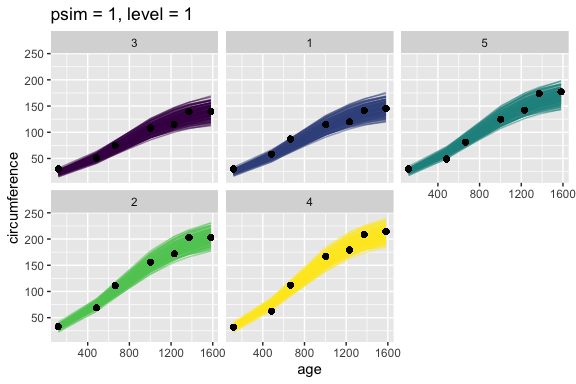Finally, the next level would be to produce intervals that would likely contain a future observation instead of just the relationship, which was captured in the figure above. The ‘bands’ below are wider than in the previous figure. The reason why the difference is small, is, because in this case the residual variance is comparatively small.

sdat21 <- simulate_nlme(fmo, nsim = 100, psim = 2, level = 1, value = "data.frame")

## Here I'm plotting points to emphasize that we are making
## predictions at the level of a single observation
ggplot(data = sdat21) +
facet_wrap(~ Tree) +
geom_point(aes(x = age, y = sim.y, color = Tree),
alpha = 0.5) +
geom_point(aes(x = age, y = circumference)) +
ggtitle("psim = 2, level = 1") +
ylab("circumference") +
theme(legend.position = "none")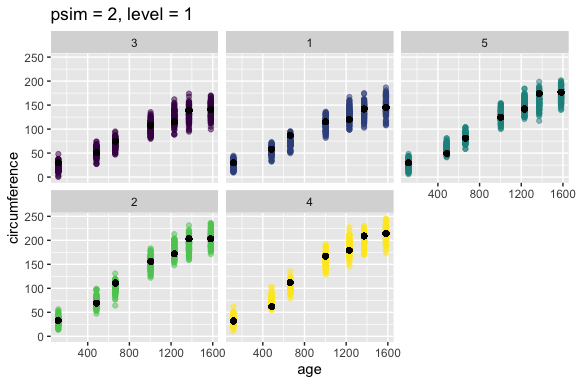The previous examples are simulations, but in order to generate confidence intervals or bands we would need to perform bootstrap, but it takes much longer, so I’m not including it in this vignette.

# Prediction

Using the previous simulation functions as a backbone it is possible to perform prediction. In this case, I’m also illustrating how to perform multimodel averaging.

## All models should be fitted using Maximum Likelihood
fm.L <- nlme(circumference ~ SSlogis(age, Asym, xmid, scal),
random = pdDiag(Asym + xmid + scal ~ 1),
method = "ML", data = Orange)
fm.G <- nlme(circumference ~ SSgompertz(age, Asym, b2, b3),
random = pdDiag(Asym + b2 + b3 ~ 1),
method = "ML", data = Orange)
fm.F <- nlme(circumference ~ SSfpl(age, A, B, xmid, scal),
random = pdDiag(A + B + xmid + scal ~ 1),
method = "ML", data = Orange)
fm.B <- nlme(circumference ~ SSbg4rp(age, w.max, lt.e, ldtm, ldtb),
random = pdDiag(w.max + lt.e + ldtm + ldtb ~ 1),
method = "ML", data = Orange)
## Let's compare the models
print(IC_tab(fm.L, fm.G, fm.F, fm.B), digits = 2)
##   model df AIC AICc BIC dAIC weight
## 1  fm.L  7 277  281 288  0.0  0.656
## 3  fm.F  9 280  287 294  2.8  0.159
## 2  fm.G  7 280  284 291  3.1  0.137
## 4  fm.B  9 282  290 296  5.3  0.047

The function predict_nlme can take one or more models and perform model averaged prediciton.

## Each model prediction is weighted according to their AIC values
prd <- predict_nlme(fm.L, fm.G, fm.F, fm.B)

ggplot(data = Orange, aes(x = age, y = circumference)) +
geom_point() +
geom_line(aes(y = predict(fm.L, level = 0), color = "Logistic")) +
geom_line(aes(y = predict(fm.G, level = 0), color = "Gompertz")) +
geom_line(aes(y = predict(fm.F, level = 0), color = "4P-Logistic")) +
geom_line(aes(y = predict(fm.B, level = 0), color = "Beta")) +
geom_line(aes(y = prd, color = "Avg. Model"), size = 1.2)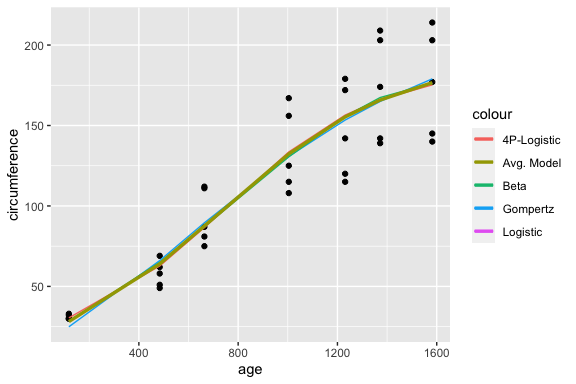And we can also compute and visualize confidence intervals.

prdc <- predict_nlme(fm.L, fm.G, fm.F, fm.B, interval = "confidence", level = 0.90)
OrangeA <- cbind(Orange, prdc)

ggplot(data = OrangeA, aes(x = age, y = circumference)) +
geom_point() +
geom_line(aes(y = predict(fm.L, level = 0), color = "Logistic")) +
geom_line(aes(y = predict(fm.G, level = 0), color = "Gompertz")) +
geom_line(aes(y = predict(fm.F, level = 0), color = "4P-Logistic")) +
geom_line(aes(y = predict(fm.B, level = 0), color = "Beta")) +
geom_line(aes(y = prd, color = "Avg. Model"), size = 1.2) +
geom_ribbon(aes(ymin = Q5, ymax = Q95), fill = "purple", alpha = 0.3)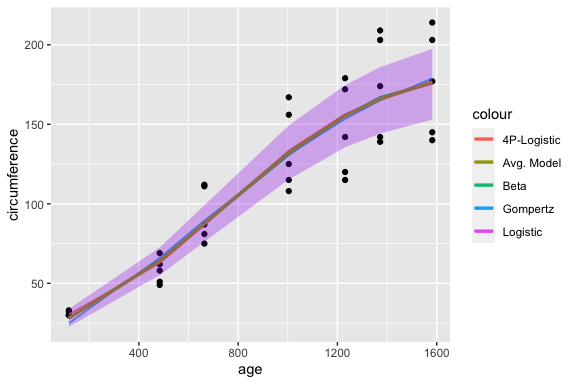# Appendix

## Note on simulate methodology

In the case of simulating data from a nonlinear model and how this relates to bootstrapping. I’ve been thinking about a number of things. In a nonlinear model, the mean function should account for a substantial part of the variability. If we think about models in terms of

$data = signal + noise$ And for mixed models: $data = fixed + random + residual$

I can see how in the linear case often the random part can be of substantial interest and sometimes more important than the fixed part, but for nonlinear models, the fixed part should dominate, otherwise we would need a better function or the data are not amenable to nonlinear modeling. In simulate_nlme, psim = 0, gives you the fitted values, so should be equivalent to ‘predict.nlme’, if theres is ever a discrepancy, then that is a bug. For psim = 1, I sample from the vector of parameters assuming that they are normally distributed. This seems to be very reasonable in practice and, in fact, much more important is the variance-covariance matrix of the vector of parameters and in particular the correlations. The problem is that in nonlinear mixed models, the relationship among parameters is not linear and can look a bit like a ‘banana’. This can be investigated through the use of bootstrap. For psim = 2, I add the residual variability which considers the modeling of the variance (heterogeneous variances), but not the possible lack of independence of the residuals. (This seems to be unlikely to arise for the type of models that we usually fit. Adding the correlation structure of the residuals can be done, but I’ll work on it when the need arises - not a priority.) It would be possible to add an options for psim = 3, where I also consider the uncertainty in the ‘random’ part above, this is is better captures, in my opinion, the ‘level’ argument in the ‘simulate_nlme’ function. By default, ‘predict.nlme’ predicts at the deepest level in the hierarchy (i.e. Q) and changing this argument allows for simulations at the different levels. Another reason, for not implementing psim = 3 (meaning sampling for the random part) is that, often, there is meaning or interest in these specific random terms. For example, sites/locations/subjects/experimenta.units are higher or lower because, in part, some characteristics that are know and some unknown, but which are out of our control. For this reason, assigning resampling or assigning a deviation at random at this level might be undesirable. If we have 10 sites labeled “A” through “J” and we know that site “A” is higher due to some characteristics (which we do not control), it would not make sense to assign the deviation from site “A” to “J” (which might be the lowest). In our analysis it might still make sense to treat ‘sites’ as random. In this case, when we use simulate_nlme, it makes more sense to use ‘psim = 2, level = 1’ (‘site’ would be our deepest level in the hierarchy - like ‘Tree’ in the ‘Orange’ example above) to generate data which appears similar to the data which was actually collected. It is true that this does not allow us to incorporate the uncertainty from the fact that we obtained these specific random deviates at the level of site (or Tree), but these will vary anyway because we are simulating forward from the vector of fixed parameters, which should account for the majority of the variability in these models. For this reason, I know that my approach is not perfect, but, so far, it seems to be usable. I’m thinking about Morris argument (see References) and I tested whether there is bias in the estimate of the random term variances using my method and I did not detect a bias - but I’m not resampling from the BLUPs. I’m also thinking that the non-parameteric part of my method (resampling the residuals) is somewhat trivial and implementing a fully parameteric option is feasible.